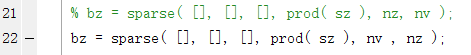# CVX error: "To RESHAPE the number of elements must not change."

Consider the following simple SDP program.

``````n=2;
t=rand(2*n,2*n);
mat=(t+t')/2;

cvx_begin SDP

variable X1(n,n) hermitian semidefinite
variable X2(n,n) hermitian semidefinite

minimize( trace(X1) + trace(X2) )
subject to

k = trace(X1) + trace(X2);
blkdiag( k*eye(n) + X1, X2 ) >= mat

cvx_end
``````

This causes the following error:

``````Error using reshape
To RESHAPE the number of elements must not change.

Error in cvx_reshape (line 52)
x = reshape( x, s );

y = cvx_reshape( y, x.size_ );

Error in cvxprob/newcnstr (line 94)

Error in  >=  (line 21)
b = newcnstr( evalin( 'caller', 'cvx_problem', '[]' ), x, y, '>=' );

Error in cvxtest (line 12)
blkdiag(k*eye(n)+X1,X2)>=mat
``````

Rewriting `blkdiag(k*eye(n)+X1,X2)>=mat` as `mat-blkdiag(k*eye(n)+X1,X2)<=0` changes the error to:

``````Matrix dimensions must agree.

Error in  +  (line 73)
bad = vx + size( remap_plus, 1 ) * ( vy - 1 );

Error in  -  (line 21)
z = plus( x, y, true, cheat );

Error in cvxtest (line 14)
mat-blkdiag(k*eye(n)+X1,X2)<=0
``````

The matrix dimensions obviously agree, so I am not sure what can be wrong. I would appreciate any help because I keep running into this problem in my code.

I am using CVX 2.1 in Matlab 2016b.

Could someone please confirm whether this is a possible bug with CVX? One can easily rewrite the problem as

``````minimize(trace(X))
subject to

X=blkdiag(X1,X2);
k=trace(X);
X+blkdiag(k*eye(n),zeros(n,n))>= mat
``````

which CVX accepts and solves, which makes me think that there is a problem with how CVX derives the dual of the problem in the original post.

Hi, there! Recently, I have come into the similar problem as you do and get the same error instructions.
After careful check and lots of experiments , I finally located the problem and solved it. It turns out that the cause of the problem is the wrongly use of the library function ‘sparse’ which is called in another library function ‘blkdiag’ .
To locate the function, firstly , you should pay much attention while checking the function ‘blkdiag’ as there maybe several functions given the same name. To save your time , I paste the path in my computer here: ‘E:\application\matlab凸优化工具箱\cvx\builtins@cvx\blkdiag’. Then check the library function ‘sparse’ which is called in ‘blkdiag’ and switch the position of last two parameters and check your problem again . In this way, your problem should be solved. The corrected function is showed below.This is great help! Thank you.